# Team:Paris Liliane Bettencourt/Project/Population counter/model

## Introduction

We aim at setting up a biological device counting random events occuring in a microfluidic device (see figure 1). These events are recombinations in the cells of the device, stimulated by pulses of arabinose coming from the tunnel. Once a certain number of pulses have been triggered, we expect our device to start emitting green fluorescence. First, we shall describe the dynamics of the population of bacteria in our device as for instance its recombinations, which further lead to the rise of the concentration of produced AHL. AHL is a quorum sensing molecule that, once a certain concentration threshold is reached, triggers in our device the production of GFP. It then alerts us of the end of counting. To count how many recombinations (so how many events) are needed to get the quorum sensing response, we shall determine the role and assess the value of a few critical parameters in our model.

## The process

To be able to model the system, we first need to have a feeling of how the components of the system react and interact with one another. First, we send a pulse of arabinose through the tunnel which triggers, with a certain probability, the recombination of some bacteria. Those bacteria are then expected to become red (RFP expression) and start producing LuxI, which in turns promotes the production of AHL. AHL will play two roles:
• it can bind with intracellular LuxR, which is a constitutively expressed protein so that its concentration inside the cell will be considered as constant. We call it LuxRf as long as it is free from AHL, LuxR* otherwise;
• it can cross the cell membrane to bind with LuxR of other bacteria in the device.

Every time a pulse of arabinose is injected, the production of AHL increases as a result of more recombinations. Once a certain level of AHL has been reached, all the cells respond to the quorum sensing signal and start producing GFP, which is the event putting an end to the counting.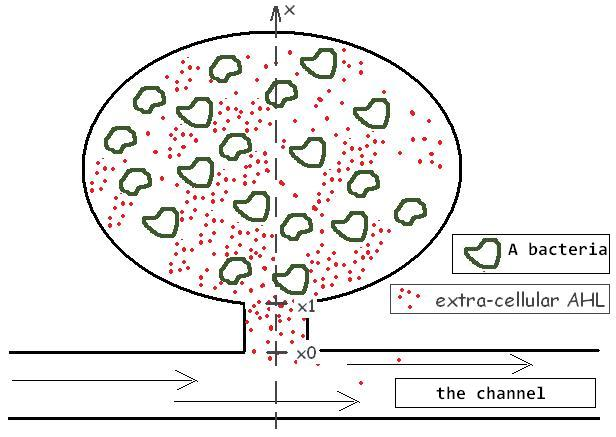Figure 1: Scheme of the microfluidic device

## The physical model

We can develop the information we have in a system of equations:
• ##### Recombination
In all our simulations, we assumed that recombination would happened after 20 minutes in contact of arabinose, plus a certain random time following an exponential law of average 10 minutes. After this random time, it is not certain that the bacteria will recombinate. In our simulations, we decided that our cell would recombinate with a probability of 0.05
• ##### LuxI
Once a bacteria has recombinated, there is necesarily a latent time before LuxI is actually synthetised. There is then some more time before a bacteria produces full rate. The amount of LuxI produced inside a bacteria stays in this very bacteria, and it is commonly accepted that the concentration of LuxI reaches a plateau and keep this value. According to the data found in the literature, we chose to set the latent time to 10 minutes. Then the concentration increases during 10 minutes linearly until the maximum concentration is reached.
• ##### LuxR
Let's call R the constant concentration of LuxR in a cell. Some of it binded with AHL and we call it LuxR*, so this gives: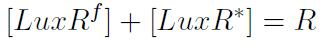The complexation between LuxR and AHL gives a chemical equilibrium determined by the constant Kreac: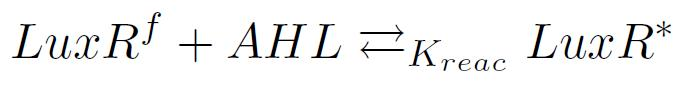so at the equilibrium: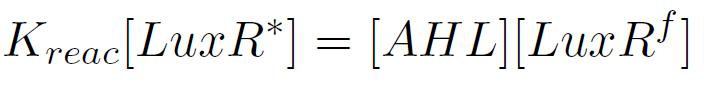which gives: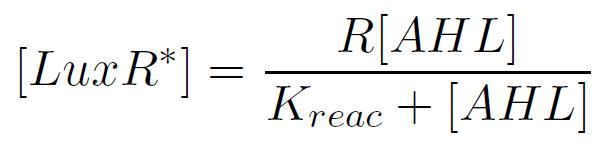• ##### AHL
Following the paper "A synchronized quorum of genetic clocks" by Hasty and al., we define the intracellular concentration of AHL, [AHLi], and the external one, [AHLe].
Internal and external AHL concentrations change as AHL is produced by the cells and can go throuh the cell membrane (kinetic constant kI and kmembrane respectively).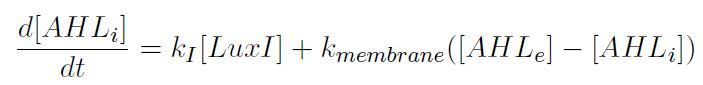The first term says that if the cell produces LuxI, then it produces AHL at a certain rate. The second term concerns the exchange between the cell and the medium, and is proportional to the difference of concentration. The concentration of AHL in the room [AHLe] decreases as the microfluidic device evacuates it, which gives birth to a diffusion term in the equation caracterized by the kinetic constant kdiff.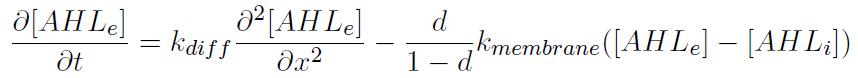where kdiff is related to parameters of the fluid and of the device, kmembrane is the same as previously, but is now compensated by d, the cell density, so that the exchanges between cells and the medium is balanced.
Here we consider only the case where the concentration of AHL is homogeneous, ie the concentration inside and outside the cell are the same. This is justified since the caracteristic time of diffusion of AHL between the outside and the inside of a cell is short compared kI and kdiff. We end up with this equation: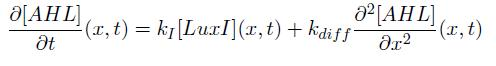In Hasty et al., kI is taken to be 0.06, which value we kept. Then, kdiff would have had to be determined experimentally. Instead, we followed Hasty et al.'s demarche, making the computations with some "reasonable" values.

### The synthesis of LuxI.

Above, we introduced directly the concentration of LuxI without giving more details. However, we need to model the production of LuxI to calibrate the counter. This means modelling how the bacteria evolve in the device and how they react to arabinose.

• Arabinose is modeled simply in the model with a diffusion equation: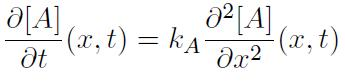with the boundary condition at the border between the device and the tunnel corresponding to: a positive value if a pulse is being sent, and 0 otherwise. Thus we get the profile of arabinose independently of everything else, except the pulse itself. kA was chosen arbitrarily (but close to values for similar molecules found in the literature) to be 10-5 mm2.min-1 . The pulse of arabinose in the channel corresponds to a concentration of 0.04%, which concentration was used in the lab.
To trigger the production of LuxI, a bacteria needs to be exposed to a minimum concentration Cc of arabinose for at least Tc minutes. For the sake of the simulation, we chose values for which we knew that recombinations would happen, here Cc = 0.001% during Tc = 1.5 min.

• Our devices have several sizes, however one device will not ever host more than 2.105 cells. We can indeed approximate the maximum number of bacteria as the ratio between the volume of a device and the volume of a cell. Moreover, considering both populations, switched and unswitched, we will consider the bacteria are in exponential growth, and their excess is evacuated through the path to the tunnel. Therefore, we need a model for the behaviour of the bacteria (movement and birth/death) that allows us to deal with a few cells (when we have a few recombinated cells for instance) up to more than 105 cells. An individually-centered model would be computationnaly inefficient, while a continuous model would perform wrong computations for small numbers of bacteria.
Birth and death happen randomly, but we know how often they happen in average. We will describe in the next section the algorithm we used to model this.
As for diffusion of bacteria, a model relying on a binomial distribution was implemented: among n cells, we pick a random number of them to move; this random number being ruled by a probability p proportional to the gradient of concentration of bacteria. Discretising time and space, one can draw at every step time a random binomial number: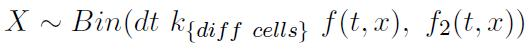where f and f2 are described in the next section.

• ## Algorithm and simulations

In this paragraph, we use several constants suggested in the paper of Hasty et al. (see references), or sometimes some approximations. We shall mention these numerical values along this paragraph.

We use a discrete lattice to make the computations, considering that the cavity of the microfluidic device can be divided in several slices of constant width dx. It has been further assumed (for simplicity of computations essentially) that diffusion only takes place along the x axis (see figure 1 above). The discrete step time wil be denoted dt. Thus our equations will depend on a one-dimensional space parameter and on time.

### Solving the diffusion equation using an implicit scheme

One can simply solve numerically a partial differential equation by discrete differentiation. The simplest way gives directly the solution at time step n+1 thanks to what we know at time n. However, this discrete explicit scheme gives a stable solution only if kdiff.dt/dx2 is less than 1. Therefore, we considered the Crank-Nicholson discretisation scheme. Unconditional to kdiff, dt and dx, this scheme produces stable computations.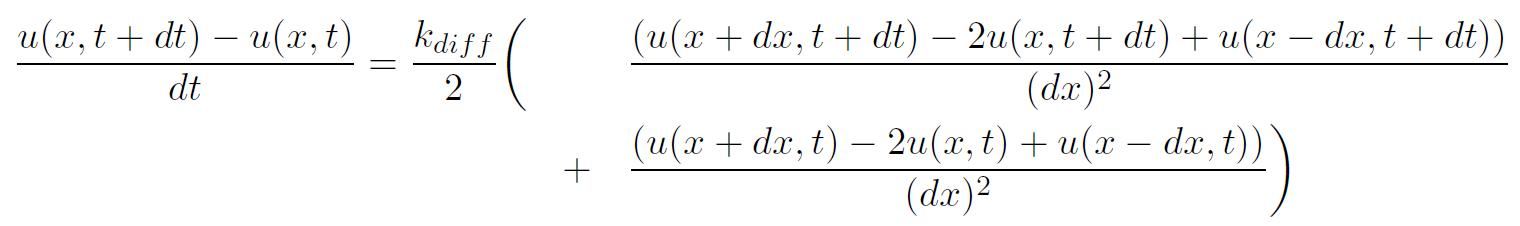However, it appears to be more complicated to solve since u(x,t+dt) cannot be obtained explicitly knowing the solution u at time t. Fortunately it can be expressed as a tridiagonal system of equations that we solved numerically.

### Stochastic Gillespie algorithm

The basic idea lying here is to compute random times for a population rather than computing them for each individual (which is much more computer intensive). Those random times appear in the recombinations and the birth-death calculus; Gillespie's idea, which we are about to describe, allows to compute the time of the next recombination (resp. birth or death) within the population instead of making the calculus and storing the result for each cell in the device.
Under the assumption that cells' recombination times are independent from one bacteria to the other, and that these times follow an exponential law of parameter λ, Gillespie tells us that the next recombination time (resp. birth or death time) follows an exponential law of parameter Npop.

### Stochastic movement of the cells

As promised before, we give the two functions describing the movement of the bacteria in the microfluidic device: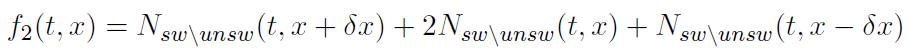where Nsw/unsw denotes either the number of switched bacteria (or recombinated bacteria) or unswitched bacteria. The idea here is to have, on average at each step time, dt.kdiff.f(x,t) bacteria that will move, a result we would get from usual diffusion. As previously mentioned, we cannot use regular diffusion since our algorithm must apply to discrete data lying on a wide range of integers.

### Simulations

Now let's present the graphical display of our simulation tool. Once the user has selected a refreshment speed x, the software will draw every x minutes the unswitched bacteria in grey, the switched ones in red and the arabinose in small blue points.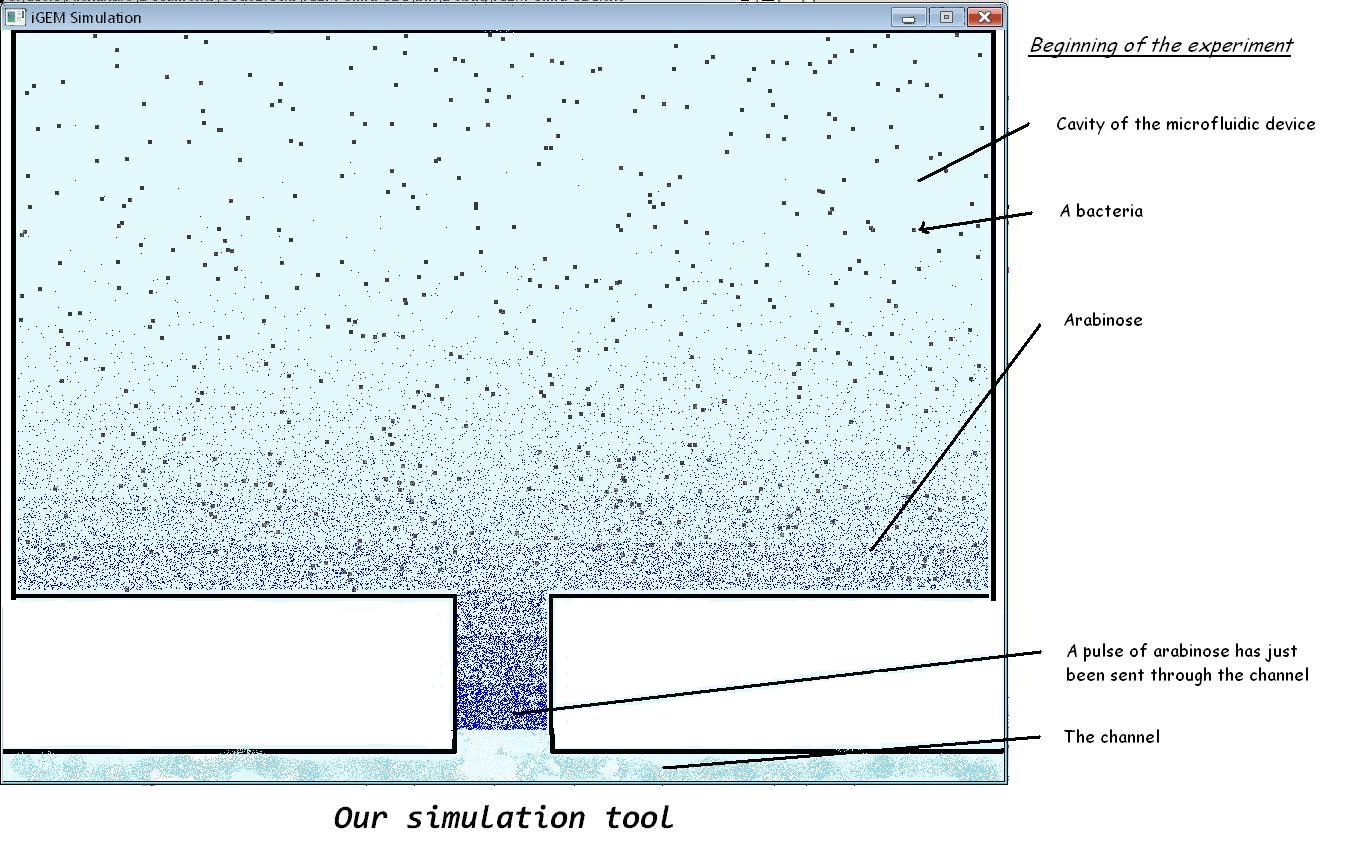We see on the following picture that after a certain amount of time, both cells and arabinose are distributed rather uniformly, and that the concentration of arabinose decreases near the tunnel as it is washed away by the flow.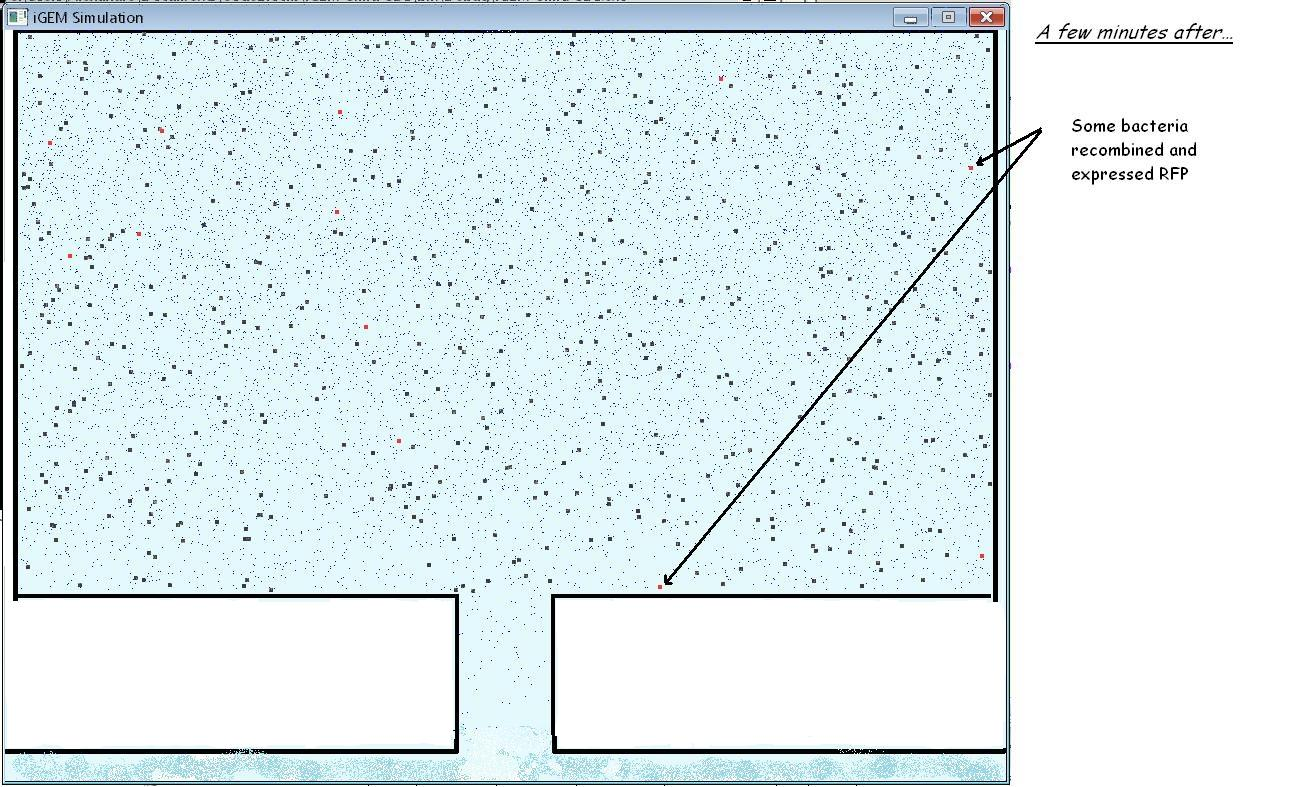The software also gives us the dynamics of recombined bacteria, LuxI and AHL slice by slice. This is what we will exploit here.
In the following simulations, the device was divided into 15 slices. We exhibit some results on the fifth slice. To count, one can either look at the number of recombined bacteria thanks to RFP, or set up the timer thanks to AHL. We will see that depending on the parameters, one or other may not be possible. For the first one, it is necessary to have clear steps, implying that the time between the pulses will be large enough to allow the system to stabilise. For the second, distinct steps on the AHL graph are necessary to set up the timer. Indeed, this allows to design the bacteria in the way that they will produce GFP only once a certain treshold in AHL was reached. However, admitting that this timer is set up, then it is possible to count more frequent events even without steps as we shall see in figure 4. In the sequel, the time between two pulses will be denoted T2.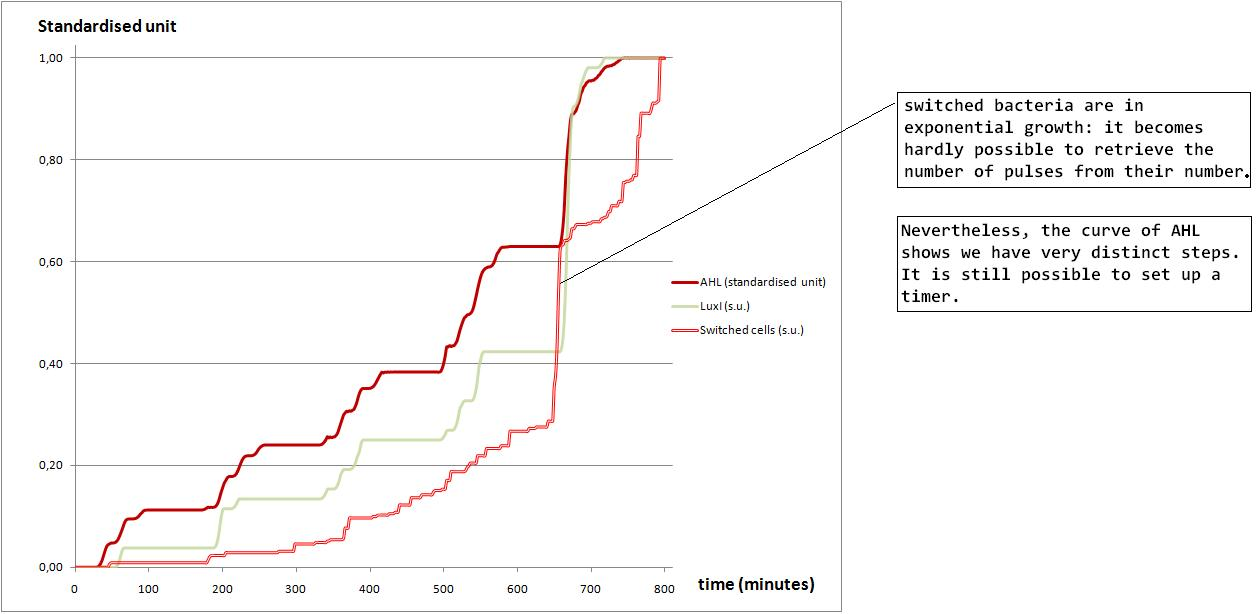Figure 2: Switched bact., AHL, LuxI for T2 = 160 and kdiff = 8.10-2

In the following figure, we show that it is still possible to count the number of pulses thanks to the number of recombined bacteria, although it might become uncertain. Nevertheless, it seems that we can still choose a threshold of AHL beyond which bacteria should be designed to luminesce. For instance, if we wanted our timer to stop at 4, then a threshold of 0.70 in standardised unit seems reasonable.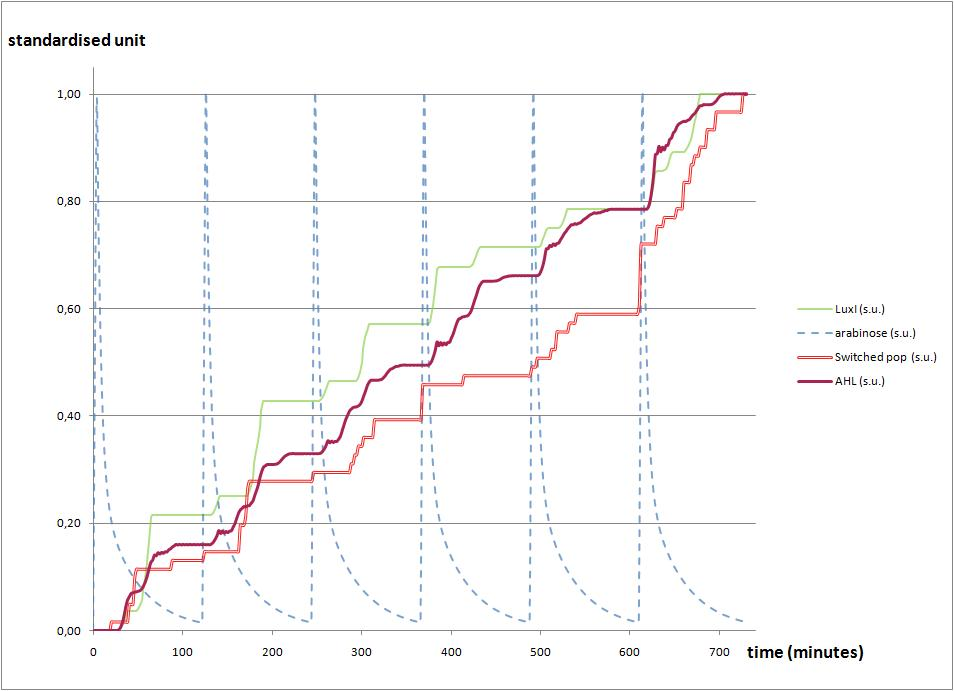Figure 3: Switched bact., AHL, LuxI for T2 = 120 and kdiff = 2.10-2

Although we have been able to count six pulses thanks to the previous graph of AHL, it is not always possible to do so. For instance, if we shorten the time between two pulses, decreasing the diffusion coefficient, then it is no longer possible to distinguish pulses: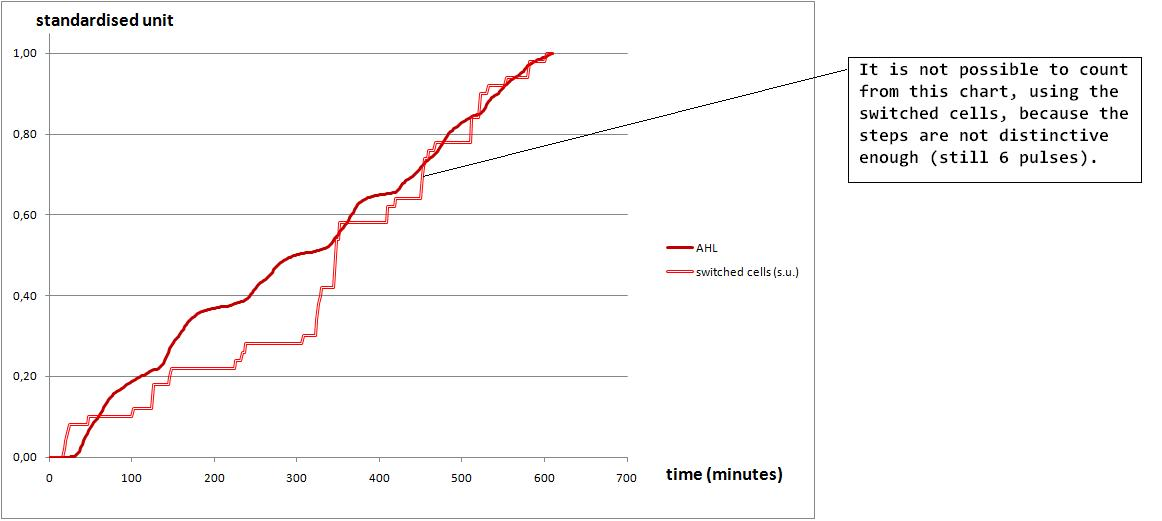Figure 4: AHL for T2 = 100 and kdiff = 10-3

## Achievements of our model

Our model gave us a better understanding of the dynamics and helped us explain and suggest experiments a posteriori. Together with the results of the experiments, we may be able:
• to have a better determination of some parameters such as caracteristic times for recombination and their statistical distribution, which we can then plug in;

• to set up parameters in a way that allow us to count with RFP, or to make a timer thanks to AHL and GFP. Those parameters are for instance the speed of the flow in the microfluidic device, the dimensions of the microfluidic device, the initial number of bacteria or the viscosity of the medium fluid.

## Sources of the simulation software

You may download the sources by clicking here. It is written in C and all the interesting parameters are in the file "constants.h". The files "nrutil.c" and "nrutil.h" are taken from the internet.

## References

• T.Danino, O.Mondragon-Palomino, L.Tsimring, J.Hasty: A synchronized quorum of genetic clocks. Nature 2009, 463:326 - 330

• T.Lu, D.Volfson, L.Tsimring, J. Hasty: Cellular growth and division in the Gillespie algorithm. Systems biology, IEEE proceedings 2004, 1:121 - 128

• D.Gillespie: A general method for numerically simulating the stochastic time evolution of coupled chemical reactions. The Journal of Computational Physics 1976, 22:403 - 434

• D.Gillespie: Exact stochastic simulation of coupled chemical reactions. The Journal of Physical Chemistry 1977, 81:2340 - 2361

• S.Lampoudi, D.Gillespie, L.Petzold: The multinomial simulation algorithm for discrete stochastic simulation of reaction-diffusion systems. 2009

• R.Bustin, H.Messer: An equivalent Markov model for Gillespie’s stochastic simulation algorithm for biochemical systems. 2006

• W.H.Press, S.A.Teukolsky, W.T.Vetterling, B.P.Flannery: Numerical recipes in C. Cambridge University Press, 1992.